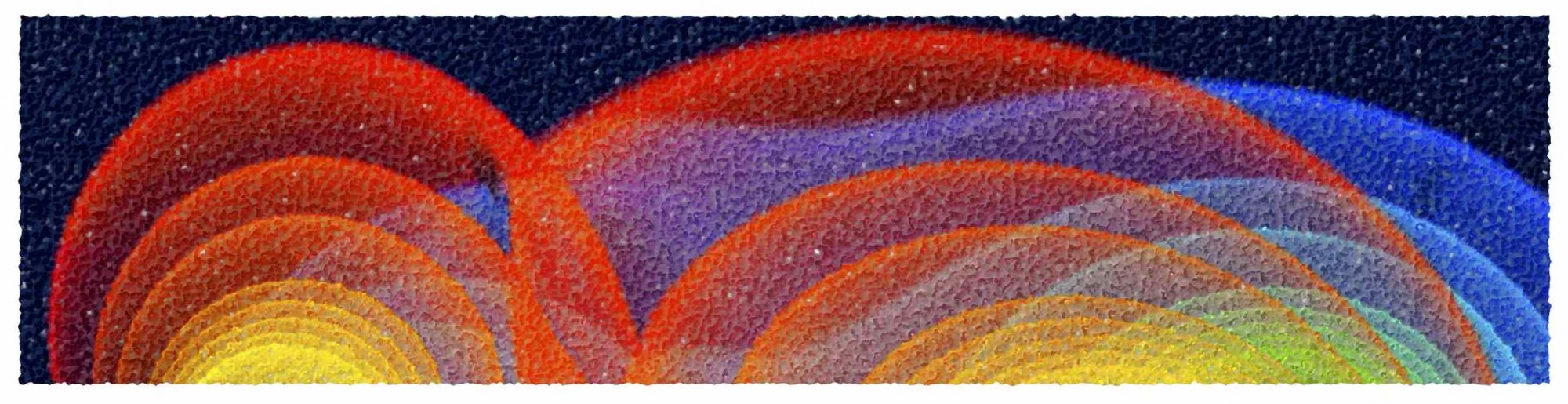# Advanced General Relativity

SS2015, Tue, 10:00-12:00, Room 2.214 (main lecture); Wed, 12:00-13:00, Room 2.216 (exercises by Dr. Galeazzi)

Assigned Exercises (solutions here). Scanned Lecture Notes

This is a course on advanced general relativity and provides an introduction to the study of solutions of Einstein equations, either in fixed or dynamical spacetimes. The course also provides an introduction to the mathematical and numerical techniques presently employed for the accurate solution of the Einstein equations together with those of relativistic hydrodynamics. The first part of the course will concentrate on the mathematical aspects of the solutions of the Einstein equations for compact objects (i.e., black holes, neutron stars), either as static/stationary solutions or when evolved (perturbation theory, gravitational collapse). A second part of the course will provide an introduction to numerical relativity, reviewing the 3+1 formulation of the equations, be it the field equations or those of relativistic hydrodynamics, the definition of hyperbolic system of partial differential equations and the development of nonlinear waves in hydrodynamics. A final part of the course,  will concentrate on the numerical aspects and the most advanced techniques for the numerical solution of these equations. The students are expected to be familiar with the theory of General Relativity and to be proficient in differential geometry and tensor calculus. A series of exercises parallels the course. A detailed syllabus and a list of references can be found here.